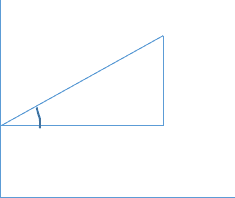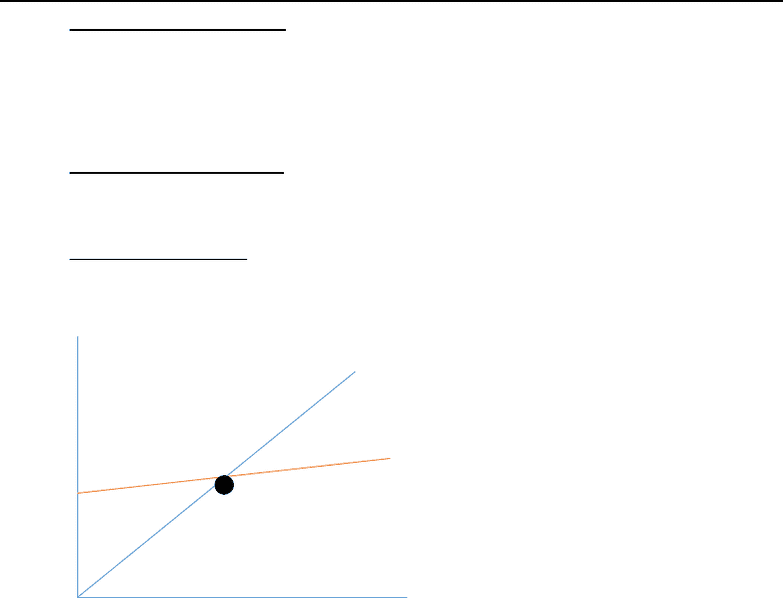Textbook Notes (280,000)
CA (170,000)
U of A (2,000)
ECON (80)
ECON102 (20)
All (7)
Chapter Lecture 11-12

# ECON102 Chapter Notes - Chapter Lecture 11-12: Government Spending, Business Cycle, Potential OutputPremium

Department
Economics
Course Code
ECON102
Professor
All
Chapter
Lecture 11-12

This preview shows pages 1-3. to view the full 9 pages of the document.
University of Alberta
ECON102
Introduction to Macroeconomics
Winter 2018
Lecture 11-12
Prof: Mesbah Sharaf
Lecture Notes

Only pages 1-3 are available for preview. Some parts have been intentionally blurred.

Subscribers OnlyChapter 9: Continuing Aggregate Supply
Review from last class: If the output in the economy increases, therefore more
employment and less unemployment. Notice the difference between employment and
There are different ways of measuring the actual GDP, there is a graphical method that
deals with the 45 degree line, Keynesian model (part of Chapter 9 Appendix)
Appendix of Chapter 9 (you must KNOW this)
Aggregate Expenditure (AE) is the measure of how much is spent on ALL final Canadian
goods and services.
AE=C+I+G+X (C: consumer expenditure, I: investment expenditures, G: government
expenditures, X: exports). Do subtract the imports from this.
Remember: C=a+bYd (follow this formula for the graph below (Figure 1)
o a: autonomous consumption
o bYd is the MPC (slope of consumption function)
o Yd: Disposable income
The angle between the a and the diagonal line here is 45 degree.
Slope= MPC
Figure 1. 45 degree concept
Diagram drawn in lecture, but created and annotated by myself
The x-axis and the y-axis are the same length
The 45 degree line is just a tool that helps us measure a variable (it measures it
vertically), this is because the horizontal distance will be equal to the vertical distance
o The 45 degree line is the actual GDP.
Since, we put “y” (which is GDP) on the horizontal axis, the 45 degree line will reflect the
“y” vertically.
a
45

Only pages 1-3 are available for preview. Some parts have been intentionally blurred.

Subscribers OnlyKeynesian Aggregate Expenditure Model
Determining equilibrium output using the 45 degree line of Aggregate Expenditure:
Above the equilibrium: When the output (Y) is greater than the Aggregate Expenditure
(AE), then there is a surplus of unsold items. The surplus items are accumulated to the
inventory. Now, sellers have lots of unsold items, the producers will call their factory,
we have lots of unsold items and please cut production, reduce it UNTIL we reach
equilibrium (where we have sold the inventory (accumulated) amount.
Below the equilibrium: When the output(Y) is less than the Aggregate Expenditure (AE),
then there is a shortage (depletion or withdraw) of unsold items/inventory. Ex: Boxing
Day. Therefore, the production by factories increases UNTIL we reach equilibrium.
At the equilibrium: Supply (Y) = Aggregate Demand (AE)
The black circle in the intersection of 45 degree line and the Aggregate
Expenditure represents the equilibrium (AE=Y)
At any output below equilibrium, the scenario is that what people want to buy is more
than the actual supply, and therefore the producers will take out products from their
inventory to meet the high demand, inventory decreases, and to recover it back to
desired level, they have to produce more.
The opposite of this would happen for a scenario that is above the equilibrium.
45 degree line: Y
what we produce
AE (Aggregate Expenditure): what